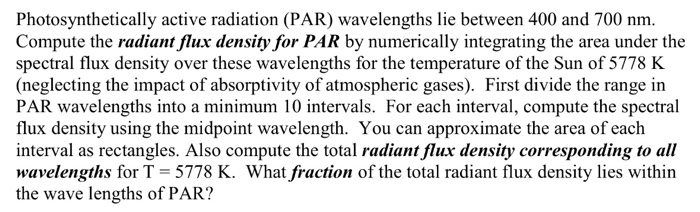# Photosynthetically active radiation (PAR) wavelengths lie between 400 and 700 nm. Compute the rad...

###### Question:Photosynthetically active radiation (PAR) wavelengths lie between 400 and 700 nm. Compute the radiant flux density for PAR by numerically integrating the area under the spectral flux density over these wavelengths for the temperature of the Sun of 5778 K (neglecting the impact of absorptivity of atmospheric gases). First divide the range in PAR wavelengths into a minimum 10 intervals. For each interval, compute the spectral flux density using the midpoint wavelength. You can approximate the area of each interval as rectangles. Also compute the total radiant flux density corresponding to all wavelengths for T 5778 K. What fraction of the total radiant flux density lies within the wave lengths of PAR?

#### Similar Solved Questions

##### At the surface of a certain planet, the gravitational acceleration g has a magnitude of 13.4...
At the surface of a certain planet, the gravitational acceleration g has a magnitude of 13.4 m/s2. A 14-kg brass ball is transported to this planet. What is the mass of the brass ball on the planet, in kg? Give the answer to the nearest whole number....
##### In what way do XHTML frame set documents differ from XHTML Web page documents?
In what way do XHTML frame set documents differ from XHTML Web page documents?...
##### We have x,y,t inRR such that x+y+t=2, xy+yt+xt=1.How to prove that x,y,t in[0,4/3]?
We have x,y,t inRR such that x+y+t=2, xy+yt+xt=1.How to prove that x,y,t in[0,4/3]?...
##### How do you find the radius of the circle: x^2 + y^2 + 12x - 2y + 21 = 0?
How do you find the radius of the circle: x^2 + y^2 + 12x - 2y + 21 = 0?...